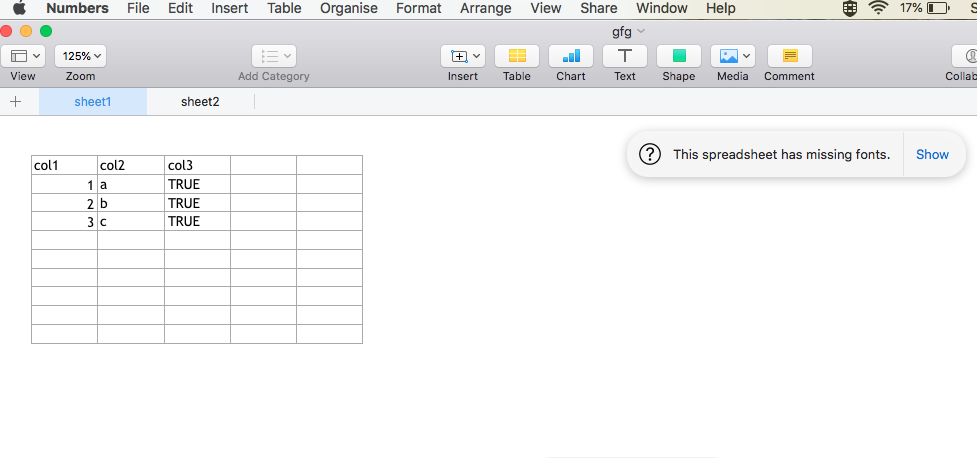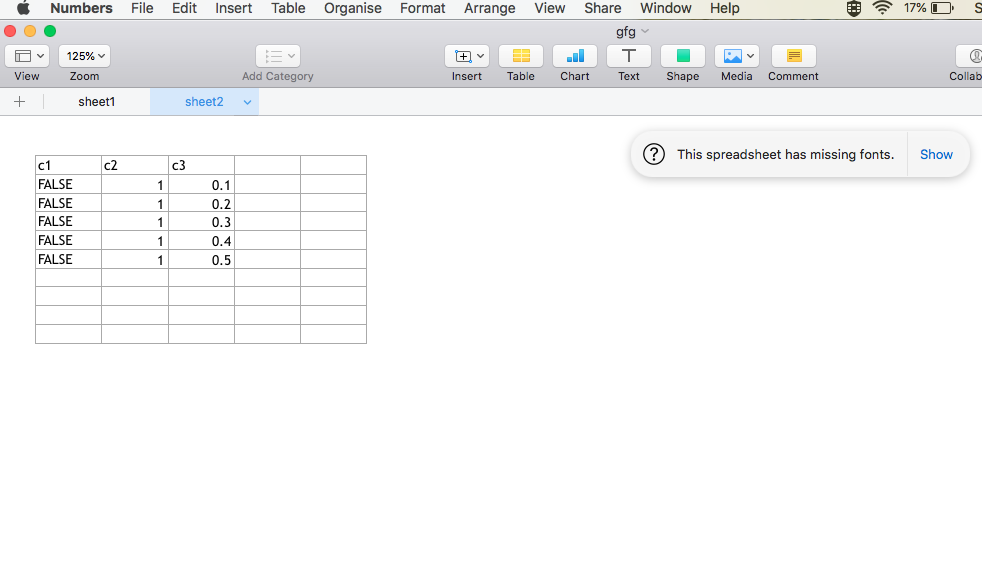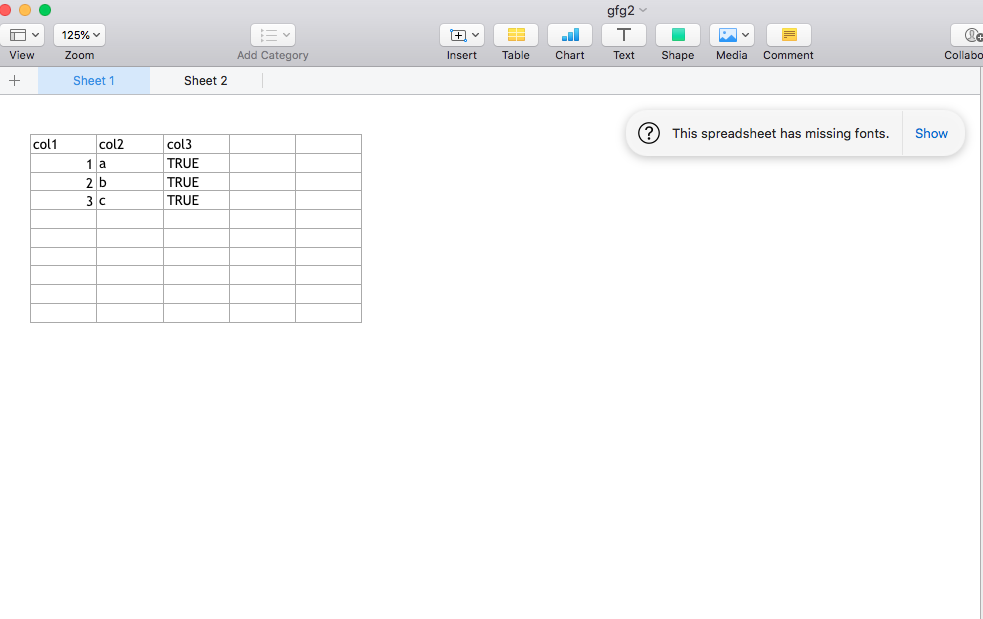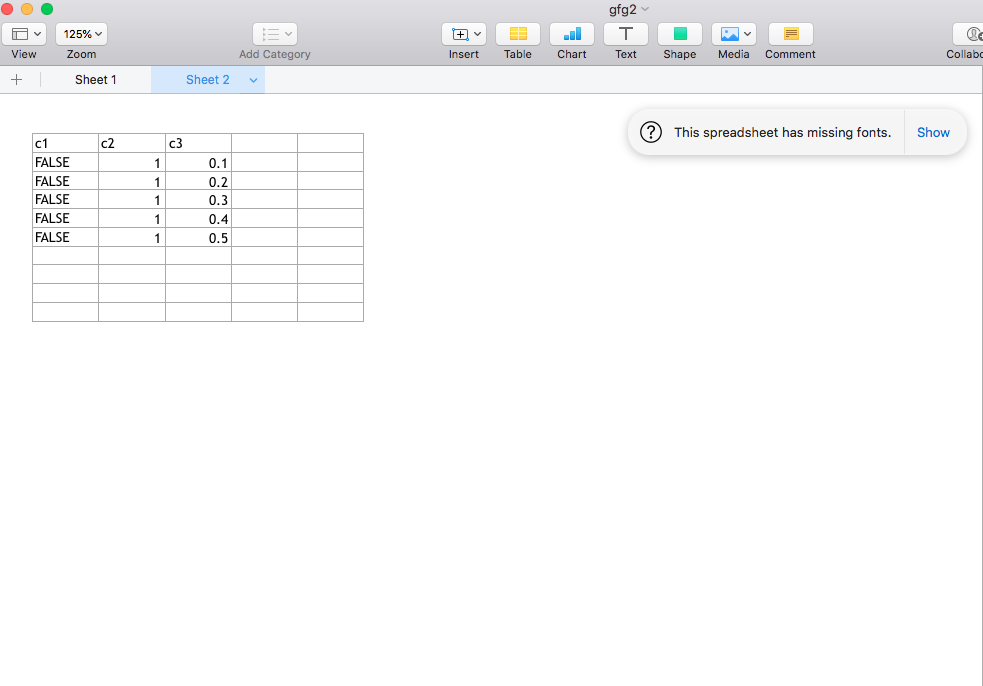# How to Export Multiple Dataframes to Different Excel Worksheets in R

• Last Updated : 17 Jun, 2021

In this article, we are going to see how to export multiple dataframe to different Excel Worksheets in R Programming Language.

We will be using the xlsx module. The xlsx library provides R functions to read/write/format Excel files and their formats. The xlsx package gives programmatic control of Excel files. The modification of the sheet’s components like the rows, columns, and cells can be carried out as well as the sheet fonts, colors and texts can be formatted. The contents of the file can be read into a data frame and the data frame can also be appended to the sheet. The package can be installed into the working space using the following syntax in R :

`install.packages ("xlsx")`

Method 1: Using write.xlsx()

The write.xlsx() method in R can be used to write a data frame into an Excel workbook. In case the R object specified as the first argument of the method is not a data frame, it can be converted to one.

Syntax: write.xlsx(df, file, sheetName = “Sheet1”, col.names = TRUE, row.names = TRUE, append = FALSE, showNA = TRUE, password = NULL )

Arguments :

• df – the data.frame to write in the workbook.
• file – the path to the output file.
• sheetName – a name assigned to the sheet.
• col.names – indicator of whether the column names of df should be written on the file.
• row.names –  indicator of whether the row names of df should be written on the file.
• append – a logical value indicating if df should be appended to an existing file.

The following code snippet creates multiple data frames and then adds them to different sheets of the same Excel sheet using the write.xlsx() method, with the specified file path name. In the case, of the second data frame, the append attribute should be equated to a TRUE value, to add the subsequent data frame to the same sheet.

## R

 `# importing required library``library``(``"xlsx"``)`` ` `# creating data frame``df1 <- ``data.frame``(col1 = ``c``(1:3),``                  ``col2 = ``letters``[1:3],``                  ``col3 = ``TRUE``)``print ``(``"Dataframe 1"``)``print ``(df1)`` ` `# creating another data frame``df2 <- ``data.frame``(c1 = ``FALSE``,``                  ``c2 = ``rep``(1,5),``                  ``c3 = ``seq``(0.1,length.out = 5,``                           ``by = 0.1))``print ``(``"Dataframe 2"``)``print ``(df2)`` ` `# accessing file path``file = ``"/Users/yashchauhan/Desktop/gfg.xlsx"`` ` `# writing contents to xlsx sheet``write.xlsx``(df1, file = file, sheetName = ``"sheet1"``,``           ``row.names = ``FALSE``)`` ` `write.xlsx``(df2, file= file, sheetName = ``"sheet2"``,``           ``append = ``TRUE``, row.names = ``FALSE``)`

Output:

``` "Dataframe 1"
col1 col2 col3
1    1    a TRUE
2    2    b TRUE
3    3    c TRUE
 "Dataframe 2"
c1 c2  c3
1 FALSE  1 0.1
2 FALSE  1 0.2
3 FALSE  1 0.3
4 FALSE  1 0.4
5 FALSE  1 0.5```Method 2: Using createWorkbook()

createWorkbook() method in R is used to create an empty workbook object. It returns a java object which contains the reference to an empty object.

`createWorkbook(type = "xlsx")`

createSheet() method in R returns the created sheet object. The workbook object can be used to invoke the createSheet() call.

`createSheet(wb, sheetName)`

The addDataFrame() method in R can be used to add a data frame to a sheet, allowing for different column styles. This method is better than the previous because it is used to customise rows and columns as well.

Syntax: addDataFrame( df , sheetName, col.names = TRUE, row.names = TRUE, startRow = 1, startColumn = 1)

Arguments :

• df – the data.frame to write in the workbook.
• sheetName – a name assigned to the sheet.
• col.names – indicator of whether the column names of df should be written on the file.
• row.names –  indicator of whether the row names of df should be written on the file.
• startRow – starting row indicator.
• startColumn – starting column indicator.

The workbook can then be saved using the saveWorkbook() method, which has the following syntax :

saveWorkbook(wb, file, password = NULL)

Code:

## R

 `# importing required library``library``(``"xlsx"``)`` ` `# creating data frame``df1 <- ``data.frame``(col1 = ``c``(1:3),``                  ``col2 = ``letters``[1:3],``                  ``col3 = ``TRUE``)``print ``(``"Dataframe 1"``)``print ``(df1)`` ` `# creating another data frame``df2 <- ``data.frame``(c1 = ``FALSE``,``                  ``c2 = ``rep``(1,5),``                  ``c3 = ``seq``(0.1,length.out = 5,by=0.1))``print ``(``"Dataframe 2"``)``print ``(df2)`` ` `# accessing file path``file = ``"/Users/yashchauhan/Desktop/gfg2.xlsx"`` ` `# creating workbook``wb = ``createWorkbook``()``sht = ``createSheet``(wb, ``"Sheet 1"``)`` ` `# add data frame``addDataFrame``(df1, sheet=sht, startColumn = 1,``             ``row.names = ``FALSE``)`` ` `# create another sheet``sht = ``createSheet``(wb, ``"Sheet 2"``)`` ` `# add data frame``addDataFrame``(df2, sheet = sht, startColumn = 1,``             ``row.names = ``FALSE``)`` ` `saveWorkbook``(wb, file)`

Output:

``` "Dataframe 1"
col1 col2 col3
1    1    a TRUE
2    2    b TRUE
3    3    c TRUE
 "Dataframe 2"
c1 c2  c3
1 FALSE  1 0.1
2 FALSE  1 0.2
3 FALSE  1 0.3
4 FALSE  1 0.4
5 FALSE  1 0.5```My Personal Notes arrow_drop_up